« Frame column stiffness Multistorey plane frames »

Coupled one-storey plane frames

As coupled plane frames are defined the plane frames that are connected in such a way that, subject to horizontal forces, they present uniform displacements.

The crossbars of the one-bay frames illustrated in the following figure have a practically infinite moment of inertia. Both columns of the first frame have moment of inertia Ι whereas the two columns of the second have Ι and 8Ι respectively.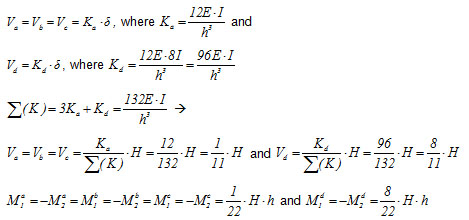In conclusion, 73% of the seismic shear force is carried by the fourth column.

Example 5.2 : Consider that the first three columns have a typical cross-section 400/400 and are 5.0 m high and that the fourth one differs only regarding one of its sides (800 mm instead of 400 mm/400, Ι 800/400 =8 Ι 400/400 ). The seismic acceleration factor is a / g =0.10.

H = 4·(0.10·800kN)= 320 kN

Va = Vb = Vc =320 ·1 /11=29.1 kN and Vd =320·8/11=232.7 kN

Ma1=-Ma2=Mb1=-Mb2= Mc1=-Mc2=29.1·5.0/2=72.8 kNm and Md1=-Md2=232.7·3.0/2=349.1 kNm

Ka=Kb=Kc= 12 · EI / h 3 =12 · 32.8 · 109 · Ν /m2 · 21.33 · 10-4m4/(5.03 · m3)=6.72·106 N / m

and

Kd=12E · 8I/h3=8· 6.72·106N/m =53.74 · 106 N/m

Σ (K)=11· 6.72·106= 73.89 · 106 N/m

Therefore,

δ = H / Σ (K)=320·103N/(73.89 · 106 N/m) =4.331 mm

In project <B_520> of the related software, the cross-section of columns C1, C2 and C3 is 400/400 and C4 is 800/400. The height of all columns is 5.0 m. The cross-section of the flanged beam is 250/500/1010/150 and its span is 5.0 m.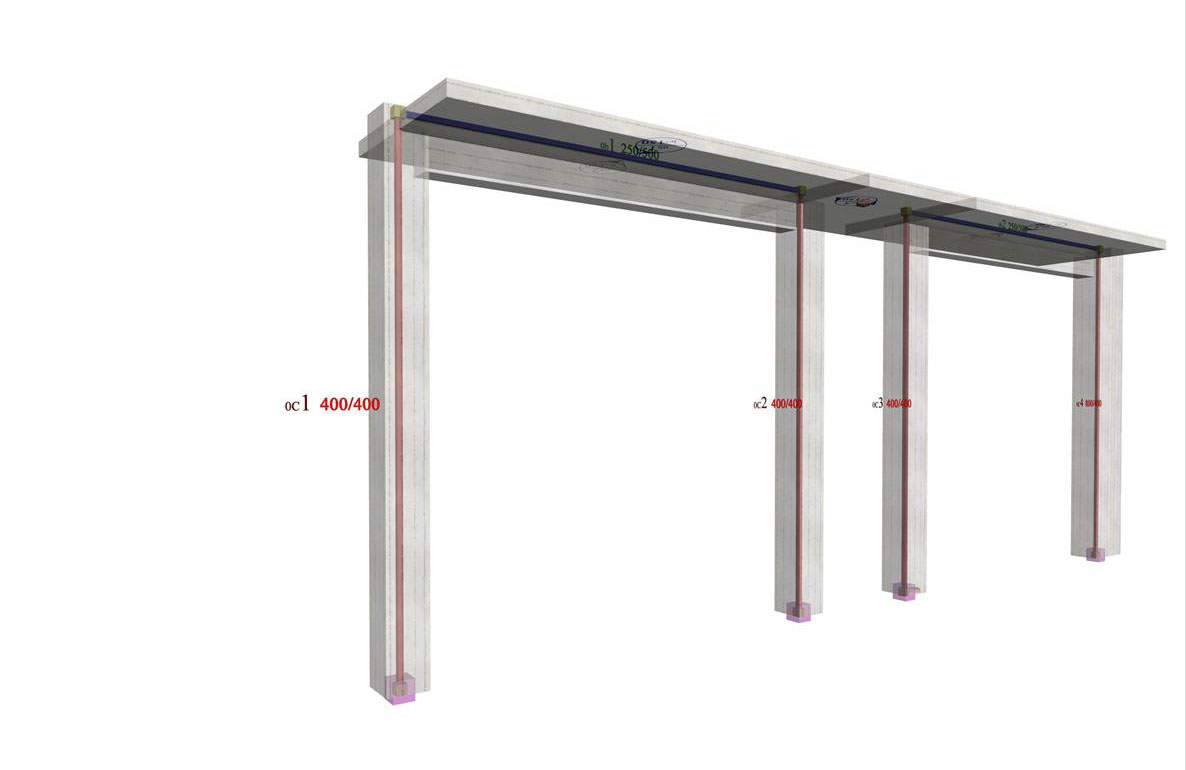Figure 5.2-2: The structural frame model fully stiffened, project <B_520>
Figure 5.2-2: The structural frame model fully stiffened, project <B_520>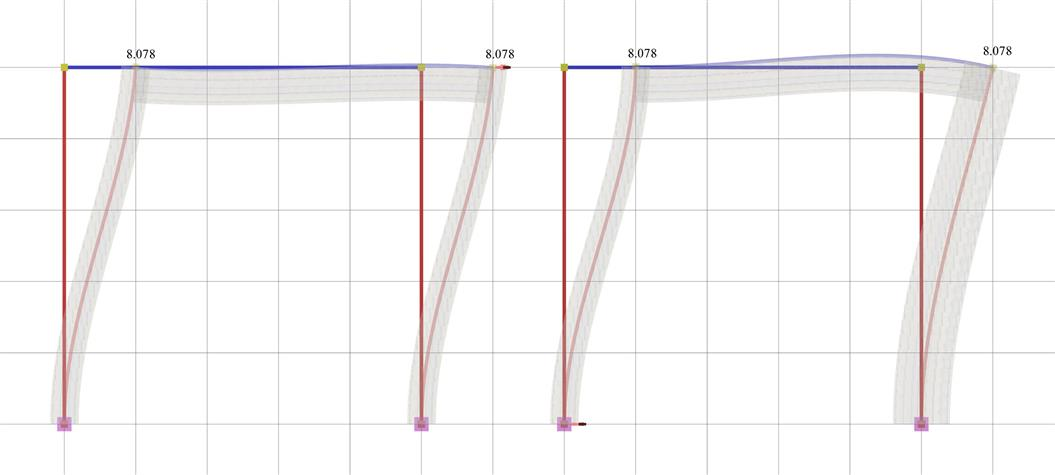Figure 5.2-3: Elastic line, δ=8.078mm Figure 5.2-3: Elastic line, δ=8.078mm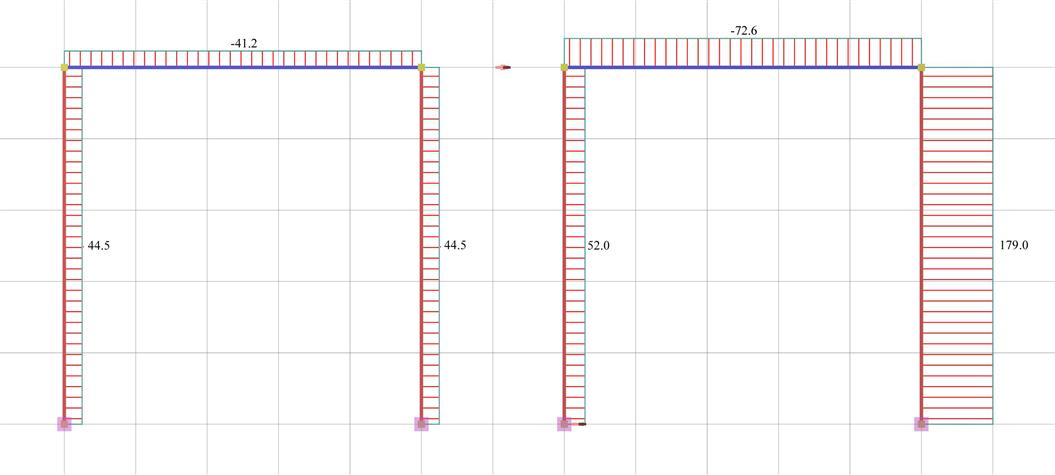Figure 5.2-4: Shear force diagrams Figure 5.2-4: Shear force diagrams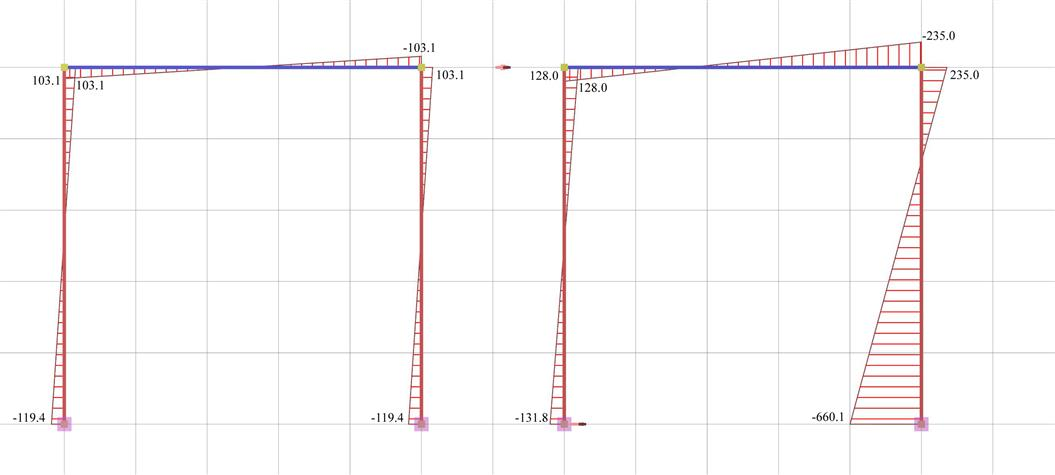Figure 5.2-5: Bending moment diagrams Figure 5.2-5: Bending moment diagrams

It should be noted that the actual displacement of the crossbar δ=8.078 mm is almost twice the value of the corresponding theoretical value δ=4.331 mm assuming fixed end conditions. This is mainly due to the strong column and the normal beam, which results in a significantly smaller actual stiffness.

The actual stiffness of columns C1, C2

Ka=Kb=Va/ δ =44.5·103N/0.008078=5.51·106 kN/m

as well as the actual stiffness of column C3

Kc=Vc/ δ =52.0·103N/0.008078m=6.44·106 kN/m

differ slightly from the corresponding value (6.72·106 N/m) of the fixed-ended column.

On the contrary, the actual stiffness of column C4

Kd=Vd/ δ =179.0·103N/0.008078m=22.16·106 kN/m

differs significantly from the corresponding value (53.74·106 N/m) of the fixed-ended column.

« Frame column stiffness Multistorey plane frames »